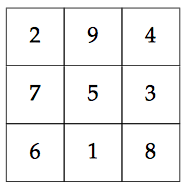How to perform Magic Square Operation in a Matrix using Python3

In this Python tutorial, we are going to learn how to perform a magic square operation in a matrix in Python. Here we will show you an easy example so that you can understand this tutorial easily.

MAGIC SQUARE OPERATION IN PYTHON

A Magic Square is:

• The square is itself having smaller squares (same as a matrix) each containing a number.
• The numbers in each vertical, horizontal, and diagonal row add up to the same value.
• The dimension of the square matrix is an (odd integer x odd integer) e.g., 3×3, 5×5, 7×7.

An example of this is given below in the image, where the sum is 15 for every column or row.magic square

To know about this interesting puzzle, Magic Square on Wikipedia

Now, let’s take a look at the code.

PROGRAM: Python program for magic square operation

#Function
def generateSquare(n):
# 2-D array with all
# slots set to 0
magicSquare = [[0 for x in range(n)]
for y in range(n)]
# initialize position of 1
i = n / 2
j = n - 1
# Fill the square by placing values
num = 1
while num <= (n * n):
if i == -1 and j == n: # 3rd condition
j = n - 2
i = 0
else:
# next number goes out of
# right side of square
if j == n:
j = 0
# next number goes
# out of upper side
if i < 0:
i = n - 1
if magicSquare[int(i)][int(j)]: # 2nd condition
j = j - 2
i = i + 1
continue
else:
magicSquare[int(i)][int(j)] = num
num = num + 1
j = j + 1
i = i - 1 # 1st condition
# Printing the square
print ("Magic Square for n =", n)
print ("Sum of each row or column",n * (n * n + 1) / 2, "\n")
for i in range(0, n):
for j in range(0, n):
print('%2d ' % (magicSquare[i][j]),end = '')
# To display output
# in matrix form
if j == n - 1:
print()
# Driver Code
# Works only when n is odd
n=int(input("Number of rows of the Magic Square:"))
generateSquare(n)

OUTPUT 1:

Number of rows of the Magic Square:7
Magic Square for n = 7
Sum of each row or column 175.0

20 12  4 45 37 29 28
11  3 44 36 35 27 19
2 43 42 34 26 18 10
49 41 33 25 17  9  1
40 32 24 16  8  7 48
31 23 15 14  6 47 39
22 21 13  5 46 38 30

OUTPUT 2:

Number of rows of the Magic Square:5
Magic Square for n = 5
Sum of each row or column 65.0

9  3 22 16 15
2 21 20 14  8
25 19 13  7  1
18 12  6  5 24
11 10  4 23 17

1.Steve Shambles says: